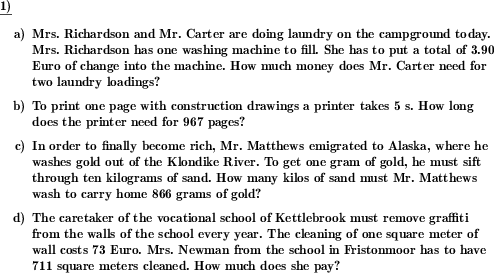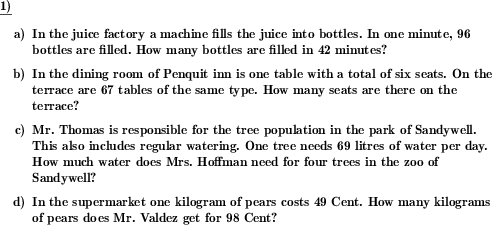Custom math worksheets at your fingertips# Details for problem "Rule of three word problem, easy variant"

Quickname: 8130

Elementary School, Primary School, Junior High School, Middle School.

## Summary

Easy word problems that can be solved by applying the rule of three. No initial division necessary, first factor is one.

## Examples## Description

Very simple word problem with simple proportional assignments.

For these problems, the proportionality constant is easily determined as the first factor it is to be multiplied with is a one. There is no need to perform a division first.

The maximum size of the proportionality constant can be set. For tasks involving money this concerns the number of cents of amounts, for tasks involving time this is the maximum number of seconds. The maximum size of the target multiplier (by which you have to multiply to get the value you are looking for) can also be limited.

Since the conversion from cents to euros may be an additional challenge, it can be determined whether such tasks are allowed.

Furthermore, the number of problems to be set can be selected.

Download free printable worksheets for this math problem here. The worksheet contains the problems only, the solution sheet includes the answers. Just click on the respective link.

•Worksheet 1Solution sheet with answers
•Worksheet 2Solution sheet with answers
•Worksheet 3Solution sheet with answers

If you can not see the solution sheets for download, they may be filtered out by an ad blocker that you may have installed. If this is the case, please allow ads for this page and reload the page. The solution sheets will then reappear.

• Do these sample worksheets do not really fit?
• Do you need more math worksheets, with a different level of difficulty?
• Would you like to combine different problems on a worksheet and adjust them to your needs?
• As a teacher, you can put together your own worksheets using the automatically generated math problems provided.
With a free initial credit, you can start creating your own math worksheets in a few minutes.

You can try it for free! Register here, to create custom worksheets now!

## Customization options for this problem

Parameter
Possible values
Number of problems
1, 2, 3, 4
Whole numbers only
Yes, No
Convert units
Never, May, Always

## Similar problems

Remark
Description
General rule of three problems with tabular representation
Apply the rule of three for a direct proportionality relationship and fill in missing values in a table.
As true rule of three problem, where the proportionality constant is calculated by division
Word problems that can be solved by applying the rule of three.